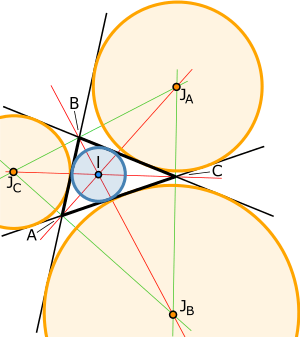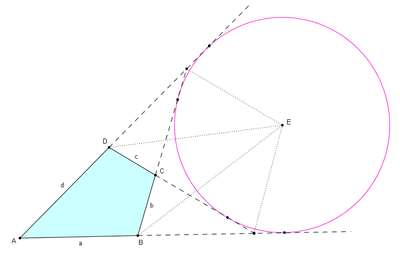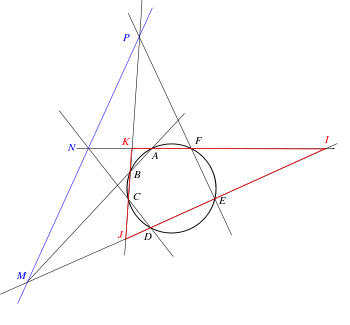# Extended sideEach of a triangle's excircles (orange) is tangent to one of the triangle's sides and to the other two extended sides.

In plane geometry, an extended side or sideline of a polygon is the line that contains one side of the polygon. The extension of a side arises in various contexts.

## Triangle

In an obtuse triangle, the altitudes from the acute angled vertices intersect the corresponding extended base sides but not the base sides themselves.

The excircles of a triangle, as well as the triangle's inconics that are not inellipses, are externally tangent to one side and to the other two extended sides.

Trilinear coordinates locate a point in the plane by its relative distances from the extended sides of a reference triangle. If the point is outside the triangle, the perpendicular from the point to the sideline may meet the sideline outside the trianglethat is, not on the actual side of the triangle.

In a triangle, three intersection points, each of an external angle bisector with the opposite extended side, are collinear.:p. 149

In a triangle, three intersection points, two of them between an interior angle bisector and the opposite side, and the third between the other exterior angle bisector and the opposite side extended, are collinear.:p. 149An ex-tangential quadrilateral ABCD and its excircle

An ex-tangential quadrilateral is a quadrilateral for which there exists a circle that is tangent to all four extended sides. The excenter (center of the tangent circle) lies at the intersection of six angle bisectors. These are the internal angle bisectors at two opposite vertex angles, the external angle bisectors (supplementary angle bisectors) at the other two vertex angles, and the external angle bisectors at the angles formed where the extensions of opposite sides intersect.

## HexagonThe intersections of the extended opposite sides of inscribed hexagon ABCDEF lie on the blue Pascal line MNP. The hexagon's extended sides are in gray and red.

Pascal's theorem states that if six arbitrary points are chosen on a conic section (i.e., ellipse, parabola or hyperbola) and joined by line segments in any order to form a hexagon, then the three pairs of opposite sides of the hexagon (extended if necessary) meet in three points which lie on a straight line, called the Pascal line of the hexagon.

## References

1. Johnson, Roger A., Advanced Euclidean Geometry, Dover Publ., 2007 (orig. 1929).